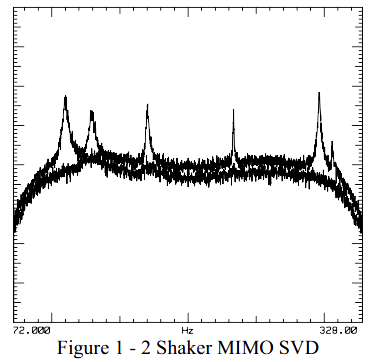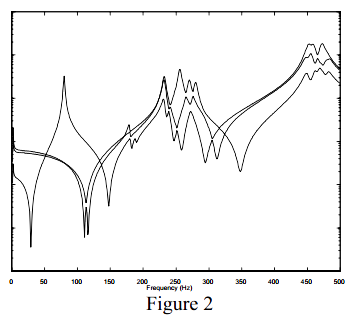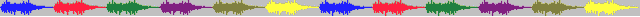# 18.模态空间00.12 — 我一直听说SVD，您能为我简单介绍一下吗？

### 模态空间 – 在我们自己的小世界  Pete Avitabile(著)  KSI科尚仪器董书伟（译）#### 我一直听说SVD，您能为我简单介绍一下吗？当然可以…

$\left [ A \right ]=\left [ U \right ]\left [ S \right ]\left [ V \right ]^{T}$

$\left [ A \right ]=\left [ \left \{ u_1 \right \} \left \{ u_2 \right \} \left \{ u_3 \right \}\,\cdots\right ]\begin{bmatrix} s_1& & & \\ & s_2& & \\ & & s_3& \\ & & &\ddots \end{bmatrix}\left [ \left \{ v_1 \right \}^T \left \{ v_2 \right \}^T \left \{ v_3 \right \}^T\,\cdots\right ]$

$\left [ A \right ]=\left \{ u_1 \right \}s_1\left \{ v_1 \right \}^T+\left \{ u_2 \right \}s_2\left \{ v_2 \right \}^T+\left \{ u_3 \right \}s_3\left \{ v_3 \right \}^T+\,\cdots$

$u_1=\begin{Bmatrix} 1\\ 2\\ 3 \end{Bmatrix};\;s_1=1$

$\left [ A \right ]_1=\begin{bmatrix} 1 &2 &3 \\ 2&4 &6 \\ 3&6 &9 \end{bmatrix}$

$u_2=\begin{Bmatrix} 1\\ 1\\ -1 \end{Bmatrix};\;s_2=1$

$\left [ A \right ]_2=\begin{bmatrix} 1 &1 &-1 \\ 1&1 &-1 \\ -1&-1 &1 \end{bmatrix}$

$\left [ A \right ]_3=\begin{bmatrix} 2 &3 &2 \\ 3&5 &5 \\ 2&5 &10 \end{bmatrix}$

$\left [ A \right ]=\begin{bmatrix} \begin{Bmatrix} 1\\ 2\\ 3 \end{Bmatrix} & \begin{Bmatrix} 1\\ 1\\ -1 \end{Bmatrix} & \begin{Bmatrix} 0\\ 0\\ 0 \end{Bmatrix} \end{bmatrix}\begin{bmatrix} 1 & & \\ & 1 & \\ & & 0 \end{bmatrix}\begin{bmatrix} \begin{Bmatrix} 1 &2 &3 \end{Bmatrix}\\ \begin{Bmatrix} 1 &1 &-1 \end{Bmatrix}\\ \begin{Bmatrix} 0 &0 &0 \end{Bmatrix} \end{bmatrix}$

$\left [ A \right ]=\begin{Bmatrix} 1\\ 2\\ 3 \end{Bmatrix}1\begin{Bmatrix} 1 &2 &3 \end{Bmatrix}+\begin{Bmatrix} 1\\ 1\\ -1 \end{Bmatrix}1\begin{Bmatrix} 1 &1 &-1 \end{Bmatrix}+\begin{Bmatrix} 0\\ 0\\ 0 \end{Bmatrix}0\begin{Bmatrix} 0 &0 &0 \end{Bmatrix}$

SVD的一个应用是对于试验模态测试，用于MIMO数据的采集。对于所有的MIMO激振器，尽管数据采集系统可能生成不相关的力函数（线性无关），但对每个激振器，实际的激振器激振力或许不能完全无关，这是由于激振器与结构之间存在相互作用造成的。